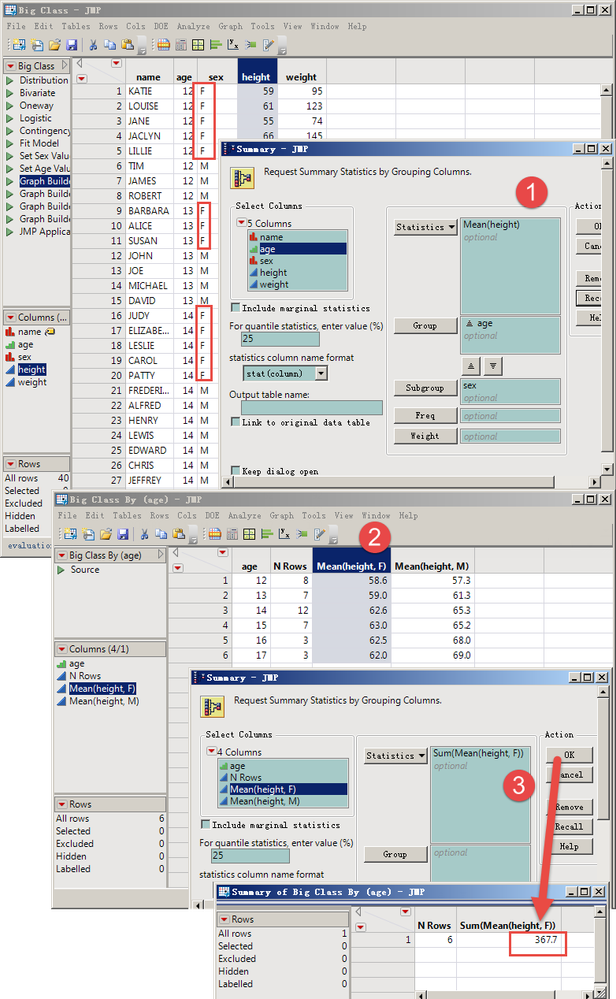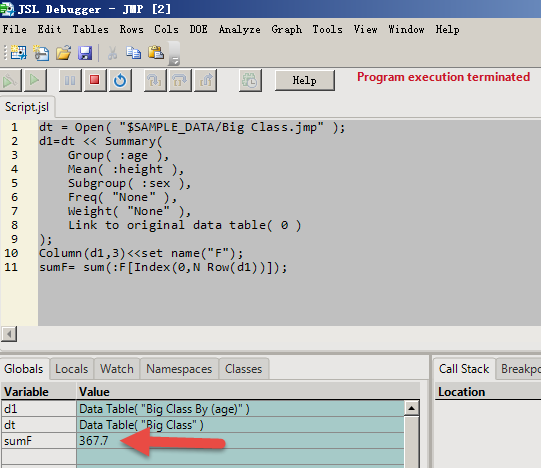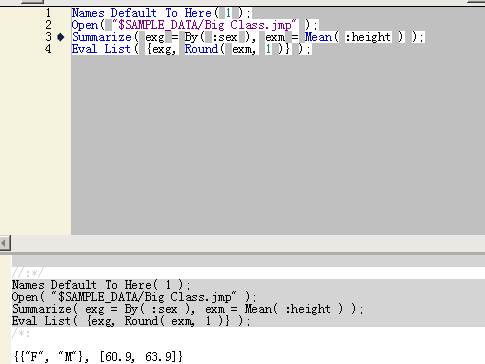Choose Language Hide Translation Bar
Highlighted

## Can the Sunmary of these steps be done in a matrix using JSL?

For example, in this file "Big Class.jmp", only the "sex"="F" is screened, the average "height" of the male is calculated for each age, and then the sum of the average height of the several.Can't appear text specification in matrix?If so, should replace the "sex"="F" with "sex"=1 first?

Thanks!

1 ACCEPTED SOLUTION

Accepted Solutions
Highlighted

## Re: Can the Sunmary of these steps be done in a matrix using JSL?

I'm not quite sure what you want to accomplish, so I'm guessing a little. If you want to avoid table operations for some reason, you may be able to take advantage of 'Summarize()'. Use 'Help > Scripting Index' for more details.

So, for example:

``````NamesDefaultToHere(1);

dt1 = Open("\$SAMPLE_DATA/Big Class.jmp");
// Because we use two 'By' variables, exg will be a list of lists.
// exm contains the mean of each group speciifed by the combination of 'By' variable levels
Summarize( dt1, exg = By( :age, :sex ), exm = Mean( :height ) );
// Sum the means for all the female groups to get 367.7
s = Sum(exm[Loc(exg, "F")]);``````
3 REPLIES 3
Highlighted

## Re: Can the Sunmary of these steps be done in a matrix using JSL?

I can only do so now, but do not know how to operate in the matrix, Thanks!As long as the sum of the average heights is obtained during the JSL run, it does not need to be displayed in the table.
Highlighted

## Re: Can the Sunmary of these steps be done in a matrix using JSL?

I'm not quite sure what you want to accomplish, so I'm guessing a little. If you want to avoid table operations for some reason, you may be able to take advantage of 'Summarize()'. Use 'Help > Scripting Index' for more details.

So, for example:

``````NamesDefaultToHere(1);

dt1 = Open("\$SAMPLE_DATA/Big Class.jmp");
// Because we use two 'By' variables, exg will be a list of lists.
// exm contains the mean of each group speciifed by the combination of 'By' variable levels
Summarize( dt1, exg = By( :age, :sex ), exm = Mean( :height ) );
// Sum the means for all the female groups to get 367.7
s = Sum(exm[Loc(exg, "F")]);``````
Highlighted

## Re: Can the Sunmary of these steps be done in a matrix using JSL?

This method should be used frequently.
Thank ian!Article Labels

There are no labels assigned to this post.